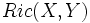Einstein tensor

This article defines a tensor (viz a section on a tensor bundle over the manifold) of type (0,2)

Definition

Given data

A manifold$M$ with a connection$\nabla$ on it. BY default, we may take a Riemannian manifold and consider the Levi-Civita connection on it.

Definition part

The Einstein tensor is a$(0,2)$-tensor that takes as input two vector fields and outputs a scalar function, as follows.$(X,Y) \mapsto Ric(X,Y) - (1/2)Rg(X,Y)$

where$Ric(X,Y)$ denotes the Ricci curvature tensor and$R$ denotes the scalar curvature function.